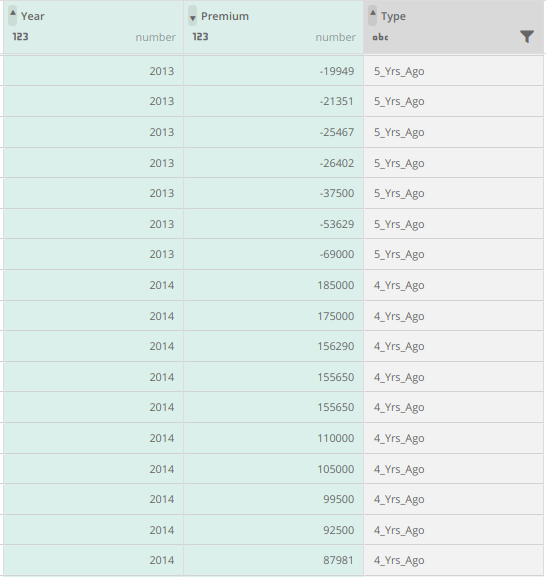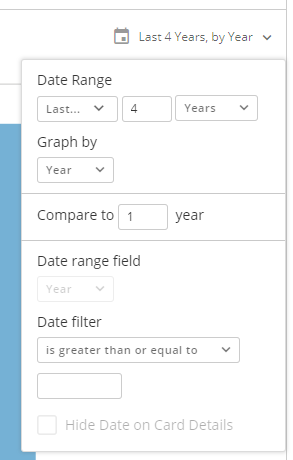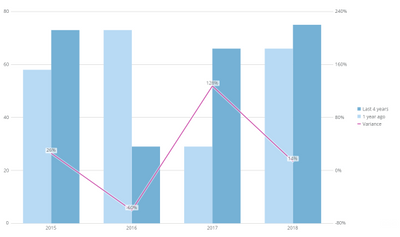%change Year over Year

Hi all,

I am trying to make a card where I will have bars for total premium by year, and one line which will show % change betwen years (current to previous, previous to two years ago etc.). Do you have any advice how to do it, I tried to find solution on Dojo and Domo support but they point me to PoP knowledge base page.

• I built a walkthrough for something similar on another post. Check out this link:

https://dojo.domo.com/t5/Card-Building/Re-Period-over-Period-using-stacked-bars/m-p/30479#M3655

Obviously instead of doing one by month, you'll need to tweak it to show years if thats what you're wanting.

For your % change calculation (starting with "Current Period / Previous Period - 1" as your Variance calculation) you'll want to build out a Case When for whatever X period you decide.

So if you're showing X1 as 2016 vs 2015 and X2 is 2016 vs 2017 you can do this:

CASE WHEN year = 2016
THEN SUM(2016 metric) / SUM (2015 metric) - 1
WHEN year = 2017
THEN SUM(2017 metric / SUM (2015 metric) - 1
END

But instead of defining a specific year, you can do DATE_SUB(CURRENT_DATE(), INTERVAL 1 year) for 2017.

I know that's a lot of info to digest, just let me know if you have any questions.

Sincerely,

Valiant

**Say "Thanks" by clicking the "heart" in the post that helped you.

• Good morning Valiant,

I am trying to implement the code you sent me into my function.

CASE WHEN `Type` = 'Current'
THEN YEAR(CURDATE())

WHEN `Type` = 'Previous_Yr'
THEN YEAR(DATE_ADD(CURDATE(), INTERVAL - 1 YEAR))

WHEN `Type` = '2_Yrs_Ago'
THEN YEAR(DATE_ADD(CURDATE(), INTERVAL - 2 YEAR))

WHEN `Type` = '3_Yrs_Ago'
THEN YEAR(DATE_ADD(CURDATE(), INTERVAL - 3 YEAR))

WHEN `Type` = '4_Yrs_Ago'
THEN YEAR(DATE_ADD(CURDATE(), INTERVAL - 4 YEAR))

WHEN `Type` = '5_Yrs_Ago'
THEN YEAR(DATE_ADD(CURDATE(), INTERVAL - 5 YEAR))

END

And then:

CASE WHEN `Type` = 'Current'
THEN (SUM(`Premium`) WHERE `Type` = 'Current') / (SUM(`Premium`) WHERE `Type` = 'Previous_Yr') - 1
WHEN `Type` = 'Previous_Yr'
THEN (SUM(`Premium`) WHERE `Type` = 'Previous_Yr') / (SUM(`Premium`) WHERE `Type` = '2_Yrs_Ago') - 1
END

But I am getting an error:
This calculation contained a syntax error.

Am I close? ?

Thank you,
Marko.

• The second statement is incorrect because of the WHERE clauses.

Can you give me a few lines of example data using your available column names? That should help me better understand what you're working with and I can tailor my answer to suit.

Sincerely,

Valiant

• I am trying to get Premium of every single row where Type is certain year. Of course, let me send you example.
Thank you,

Marko.

•• Ok, so while we could go down a long path of lots of sql transform and beast modes to get this answer, let's see if the simple solution works first.

In your data, can you edit the data (either using an ETL or SQL transform) to convert each year (ie, 2013) to something like this (2013-01-01). The end result being that we want to convert that column to a recognized date column type. Once you have that,  you can go to the card, choose the Period over Period type and select 'Variance bar line'. Using the Year as your X axis and the Premium as your Y, adjust the time selection to match the following:Once you have that, you'll have bars for each year compared to the previous year and a variance (% change) line for your chart.Let me know if that will work for your needs,

Valiant

• I already have made this one, but we would like to have one bar for each year and one line showing % change between current and previous year. That's why I am trying to find a solution for this.

Thank you very much for your help,

Marko.

• ok, so in that case, you're going to need to create to do the following steps via either ETL or SQL transforms:

FROM dataset
GROUP BY `Year`

SELECT a.*,